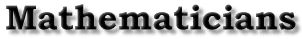| Cauchy's Life | Cauchy's Mathematical Accomplishments | Cauchy's Criterion for Convergence | Bibliography | Back to the front page Cauchy's Criterion for Convergence Somsack Chaitesipaseut Cauchy's Criterion for Convergence first appeared in Cauchy'sCours d'Analyse of 1821. Since I could not find a copy of this work, I could not make a copy of it. Thus, I had to resort to his Oeuvres Complètes for a copy of an early print of his criterion for convergence. Cauchy writes, ``il est nécessaire et il suffit que la différence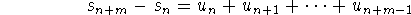devienne infiniment petite, quand on attribue au nombre n une valeur infiniment grande, quel que soit d'ailleurs le nombre entier représenté par m'' [Cauchy, p. 267]. What this means (in English and in modern terms) is that a necessary and sufficient condition for convergence of a sequence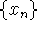is that it be a Cauchy sequence, where a Cauchy sequence is defined to be for each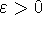, there is a positive integer N such that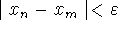for all m>N and all n>N [Protter, p. 76]. Even though this criterion for convergence bears Cauchy's name, he was not the first mathematician to derive this condition. Other mathematicians like Lacroix, Euler and da Cunha had already given similar forms for this criterion several years before Cauchy presented his. Yet, Cauchy's name stuck with this criterion. Some explain this by saying that it was because of his accompanying definitions for convergent and divergent sequences that his name was attached to this criterion over time [Katz, p.712; Grabiner, p103-104]. For the definitions of convergent and divergent, Cauchy writes, ``Let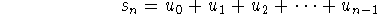be the sum of the first n terms [of a series], n designating an arbitrary integer. If, for increasing values of n, the sum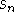approaches indefinitely a certain limit s, the series will be called convergent, and the limit in question will be called the sum of the series. On the contrary, if, as n increases indefinitely, the sumdoes not approach any fixed limit, the series will be divergent and will not have a sum'' [Katz, p. 712]. It is only after these definitions for convergent and divergent series that Cauchy was able to state his criterion for convergence. At the time, mathematicians knew that having a series which approaches zero does not necesarily mean having a converging sequence. This is where the Cauchy Criterion for Convergence comes in. Convergence could only be assured if the various sums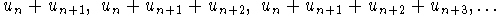``taken, from the first, in whatever number one wishes, finish by constantly having an absolute value less than any assignable limit'' [Katz, p. 712]. It is obvious that without the second statement, the sequence could be divergent. An example of this is the series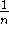for n>0 and n an integer. Note that the sequence diverges as. Looking at the Cauchy Criterion, we see that there are two parts to it. One part states what is necessary for convergence, and the other says that this is sufficient to prove convergence [Grabiner, p. 103]. Yet, Cauchy did not offer any proof for the sufficiency condition. The reason for this was that there was no arithmetical definition of the real numbers at the time, which is needed in proving sufficiency [Katz, p. 712]. What Cauchy did do was present examples where his criterion holds true. One such example is the converging geometric expression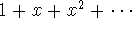for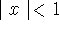. Cauchy showed, by actual computation, that this converging series satisfies the Cauchy Criterion because the finite expression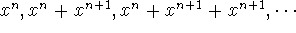were always included between the boundsand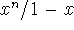[Grabiner, p. 104]. | Cauchy's Life | Cauchy's Mathematical Accomplishments | Cauchy's Criterion for Convergence | Bibliography | Back to the front page